# Vectorised Patterns in R Graphics

Support for pattern fills was added to the R graphics engine in R version 4.1.0, with an R interface via the ‘grid’ package.

library(grid)

For example, the following code defines a linear gradient that varies horizontally from red to white and a tiling pattern that is based on a repeating red circle.

gradcol <- c(palette(), "white")
patcol <- 2
pat <- pattern(circleGrob(r=unit(2, "mm"), gp=gpar(col=patcol, fill=patcol)),
width=unit(5, "mm"), height=unit(5, "mm"),
extend="repeat")

The next code calls grid.rect() twice, once to fill a rectangle with the linear gradient and a second time to fill another rectangle with the tiling pattern.

grid.rect(1/3, width=1/4, height=1/2,
grid.rect(2/3, width=1/4, height=1/2,
gp=gpar(fill=pat))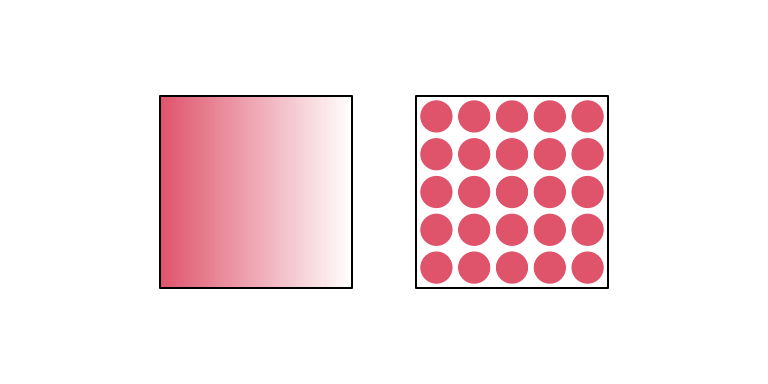The following code draws the two rectangles with a single call to grid.rect() and fills them both using the linear gradient. The result is that both rectangles are filled with the same gradient from red to white, with the gradient relative to a bounding box around both rectangles (we start with red at the left edge of the rectangle on the left and end with white at the right edge of the rectangle on the right).

grid.rect(1:2/3, width=1/4, height=1/2,
gp=gpar(fill=grad))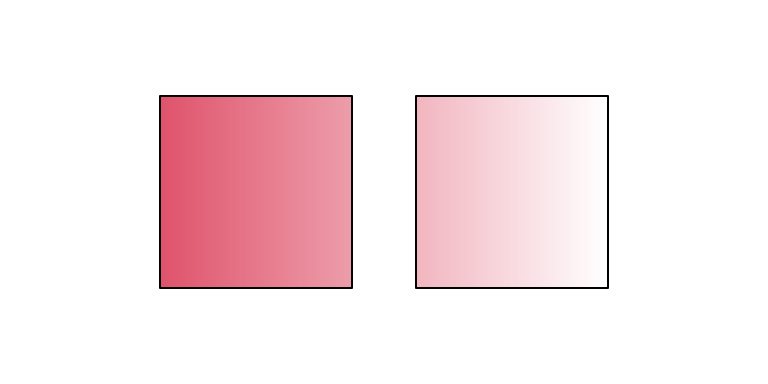From R 4.2.0, we can produce a different result because the linearGradient() function has gained a group argument. If we set this to FALSE, then we can fill both rectangles with the “same” gradient fill, but the gradient fill is relative to individual rectangles, as shown below.

grad2 <- linearGradient(gradcol, y1=.5, y2=.5,
group=FALSE)
grid.rect(1:2/3, width=1/4, height=1/2,
gp=gpar(fill=grad2))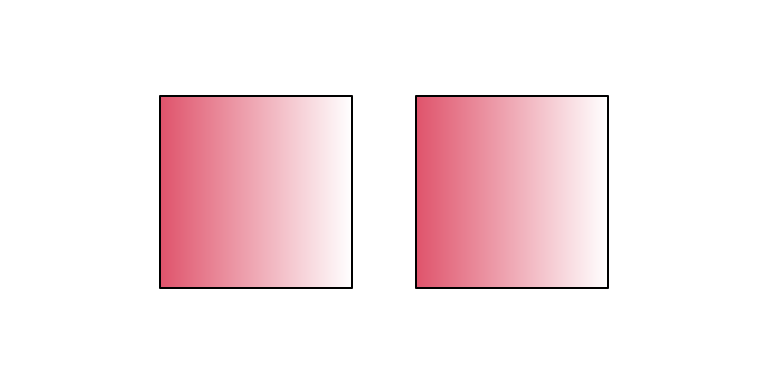Another change in R 4.2.0 is that we can specify more than one pattern fill. For example, the following code draws the two rectangles in a single grid.rect() call and provides a list of pattern fills: the original linear gradient and the tiling pattern. The result is that the first rectangle is filled with the linear gradient and the second rectangle is filled with the tiling pattern.

grid.rect(1:2/3, width=1/4, height=1/2,
gp=gpar(fill=list(grad, pat)))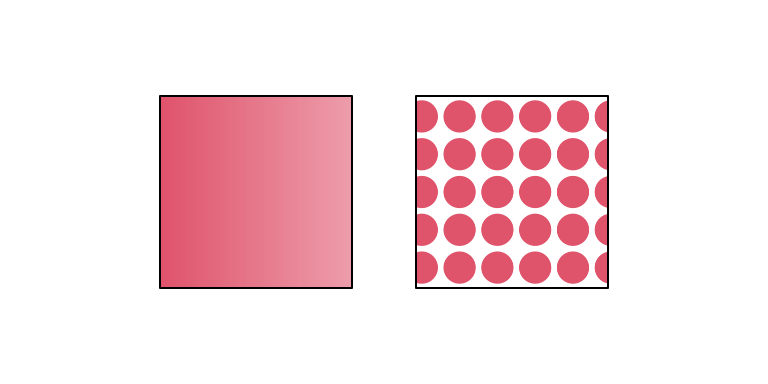The result is different from the very first example because both the linear gradient and the tiling pattern are relative to a bounding box around both rectangles. The following code uses the new group argument to fill each rectangle with a different pattern fill and have the pattern fills relative to the individual rectangles.

pat2 <- pattern(circleGrob(r=unit(2, "mm"), gp=gpar(col=patcol, fill=patcol)),
width=unit(5, "mm"), height=unit(5, "mm"),
extend="repeat",
group=FALSE)
grid.rect(1:2/3, width=1/4, height=1/2,
gp=gpar(fill=list(grad2, pat2)))A further addition in R 4.2.0 is the ability to use pattern fills on “points” (data symbols). Combining that with pattern lists and group=FALSE, we can, for example, apply pattern fills to individual points on a scatterplot, as shown below. For this example, the pattern fill is a radial gradient from white to red.

library(gggrid)
pointsGrob(coords$x, coords$y, pch=21,
mapping=aes(x=disp, y=mpg))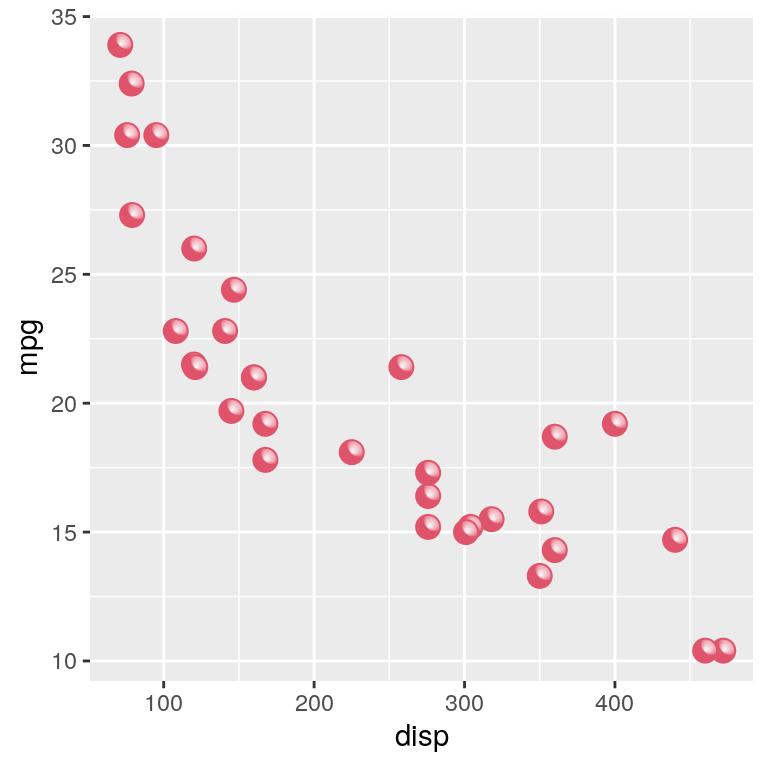Further discussion and more detail about these new facilities can be found in the technical report “Vectorised Pattern Fills in R Graphics”. The technical report “Constructive Geometry for Complex Grobs” describes related changes to the grobCoords() function that is used in the resolution of pattern fills.